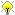[Documentation] [TitleIndex] [WordIndex]Please ask about problems and questions regarding this tutorial on answers.ros.org. Don't forget to include in your question the link to this page, the versions of your OS & ROS, and also add appropriate tags.

# Covariance Ellipsoids

Description: Algorithms for 2d and 3d covariance ellipsoids, typically used in slam.

Keywords: ecl statistics

Tutorial Level: INTERMEDIATE

Contents

## Overview

Implemented by the template class CovarianceEllipsoid - this takes two template parameters. The first is the float type used (usually float/double) and the second, the dimension of the ellipsoid to be determined.

Specialisations are enabled for 2 and 3 dimensional types only as we haven't had a need to develop a general implementation.

## Typedefs

Typedefs exist for the template class in the eigen style:

```   1 using ecl::CovarianceEllipsoid2f;
2 using ecl::CovarianceEllipsoid2d;
3 using ecl::CovarianceEllipsoid3f;
4 using ecl::CovarianceEllipsoid3d;
```

## Usage

You typically feed the covariance ellipsoid with a positive definite symmetric matrix (eigen matrix). Take care entering the positive definite symmetric matrix - the class does not yet do checking to make sure it is positive definite symmetric.

You can either input the matrix via the constructor - in which case it will automatically calculate the ellipsoid properties, or pass it in later via the compute() method:

```   1 Matrix2d M;
2 M << 3.0, 1.0, 1.0, 5.0; // must be a positive definite, symmetric matrix
3
4 CovarianceEllipsoid2d ellipse(M);
5
6 // OR
7
8 CovarianceEllipsoid2d ellipse;
9 ellipse.compute(M);
```

```   1 Matrix2d M;
2 M << 3.0, 1.0, 1.0, 5.0;
3 CovarianceEllipsoid2d ellipse(M);
4
5 // ellipse minor and major axis lengths
6 const Vector2d& lengths = ellipse.lengths();
7 double eigen_value_0 = lengths*lengths;
8
9 // angle between x-axis and major axis of the ellipse
10 double angle = ellipse.rotation();
11
12 // values of the intercepts of the ellipse with x and y axes
13 const Vector2d& intercepts = ellipse.intercepts();
14
15 // the ellipse axes/covariance eigenvectors as column vectors in a matrix
16 const Matrix2d& axes = ellipse.axes();
```

The 3d version is similar, but will only calculate intercepts and axes respectively. Note that the axes are sorted (to ensure a right-handed co-ordinate system) and normalised.

```   1 Matrix3d P;
2 double sigmaX(0.1);
3 double sigmaY(0.5);
4 double sigmaT(0.3);
5 P << sigmaX*sigmaX, 0, 0, 0, sigmaY*sigmaY, 0, 0, 0, sigmaT*sigmaT;
6 CovarianceEllipsoid3d ellipse(P);
7
8 const Vector3d& lengths = ellipse.lengths();
9 double eigen_value_0 = lengths*lengths;
10 const Matrix3d& axes = ellipse.axes();
```

2020-01-25 12:37